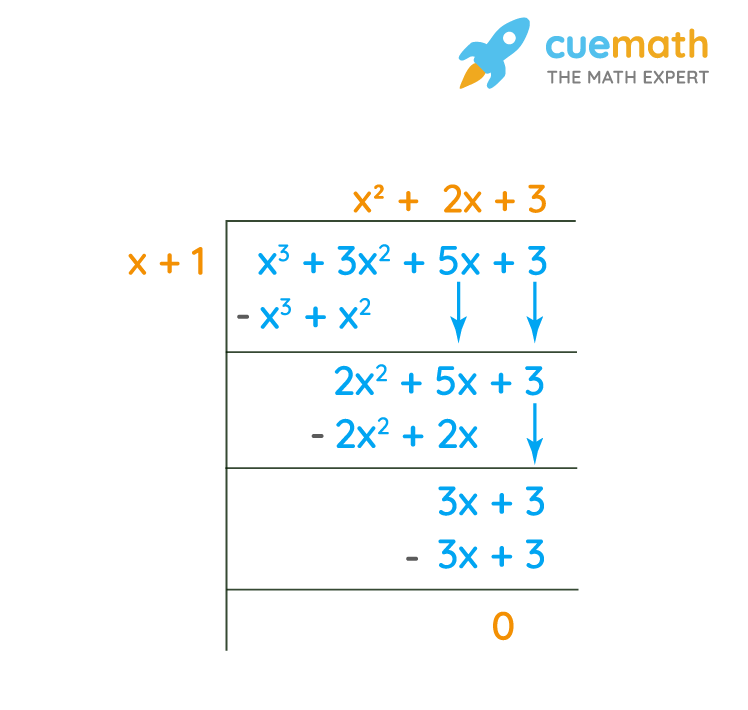# What Is the Quotient (x3 + 3x2 + 5x + 3) ÷ (X + 1)?

## Question: What is the quotient (x3 + 3x2 + 5x + 3) ÷ (x + 1)?

The quotient is defined as it is produced by the division of two numbers.

## Answer: x2 + 2x + 3

After dividing, how much it is dividing is the quotient.

## Explanation:

We will perform long division on the problem (x3 + 3x2 + 5x + 3) ÷ (x + 1)